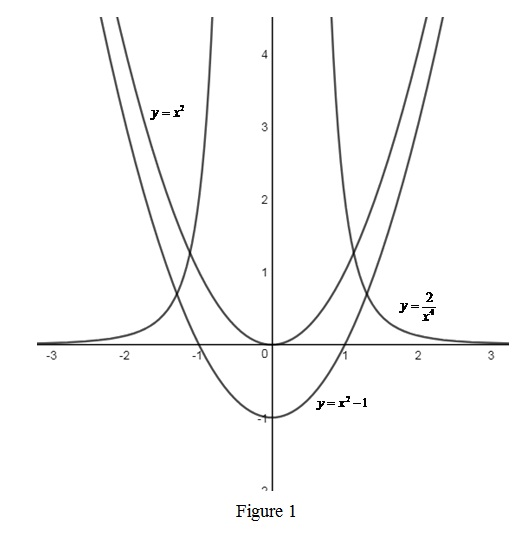# An even function; how to identify an even function by graph; three example of even function.### Single Variable Calculus: Concepts...

4th Edition
James Stewart
Publisher: Cengage Learning
ISBN: 9781337687805### Single Variable Calculus: Concepts...

4th Edition
James Stewart
Publisher: Cengage Learning
ISBN: 9781337687805

#### Solutions

Chapter 1, Problem 3RCC

(a)

To determine

## To explain: An even function; how to identify an even function by graph; three example of even function.

Expert Solution

### Explanation of Solution

Even function: A function f(x) is said to be an even function if it satisfies condition, f(x)=f(x). Each point in a negative y-axis is a reflection of the points in the positive x-axis. That is, if there exist a point (3, 4), then there also will be a point (−3, 4).

How to identify an even function: If a graph of the function is symmetric about the y-axis then the function is said to be an even function.

Examples:

Three possible even functions are y=x2, y=x21 and y=2x4.

Graphs of the above three functions are shown below in Figure 1.From Figure 1, it is observed that each graph is symmetric about the y-axis.

(b)

To determine

Expert Solution

### Explanation of Solution

Even function: A function f(x) is said to be an odd function if it satisfies condition, f(x)=f(x). The points of an odd function is in the form of (3, 4) and (−3, −4).

How to identify an even function: If a graph of the function is symmetric about the origin then the function is said to be an odd function.

Examples:

Three possible even functions are y=x3+x, y=x and y=1x3.

Graphs of the above three functions are shown below in Figure 2.From Figure 2, it is observed that each graph is symmetric about the origin.

### Have a homework question?

Subscribe to bartleby learn! Ask subject matter experts 30 homework questions each month. Plus, you’ll have access to millions of step-by-step textbook answers!